Precalculus : Solve a Triangle in Which 2 Sides and an Included Angle Are Given (SAS)

Example Questions

Example Question #34 : Trigonometric Applications

Use the Law of Cosines to find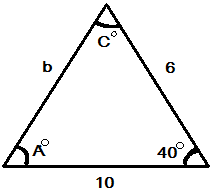(Triangle not drawn to scale.)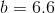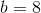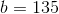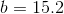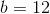Explanation:

We need to use the Law of Cosines in order to solve this problem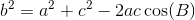in this case,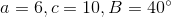In order to arrive at our answer, we plug the numbers into our formula: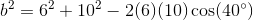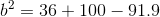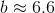Note: we use the "approximately" to indicate the answer is around 6.6. It will vary depending on your rounding.

Example Question #1 : Law Of Cosines

Use the Law of Cosines to find.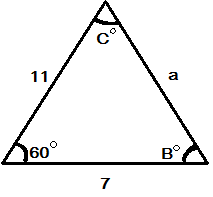(Triangle not drawn to scale.)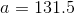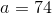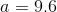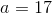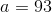Explanation:

In order to solve this problem, we need to use the following formula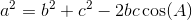in this case,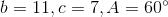We plug our numbers into our formula and get our answer: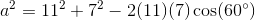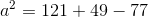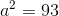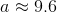Note: we use the "approximately" to indicate that the answer is around 9.6. It will vary depending on your rounding.

Example Question #421 : Pre Calculus

The 2 sides of a triangle have lengths of 10 and 20.  The included angle is 25 degrees.  What is the length of the third side to the nearest integer?Explanation:

Write the formula for the Law of Cosines.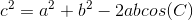Substitute the side lengths of the triangle and the included angle to find the third length.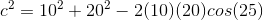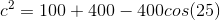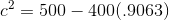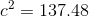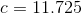Round this to the nearest integer.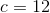Example Question #2 : Law Of Cosines

What is the approximate length of the unknown side of the triangle if two sides of the triangle areand, with an included angle of?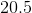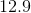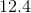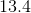Explanation:

Write the formula for the Law of Cosines.Substitute the known values and solve for.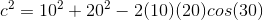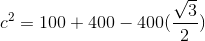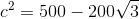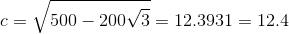Example Question #1 : Solve A Triangle In Which 2 Sides And An Included Angle Are Given (Sas)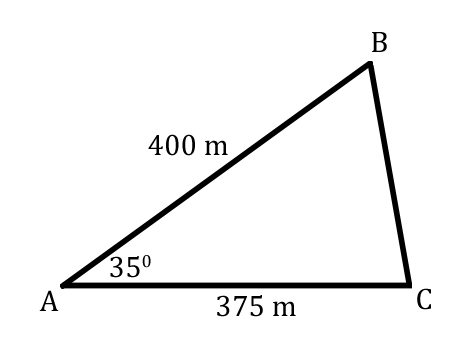What is the measurement of sideusing the Law of Cosines? Round to the nearest tenth.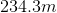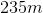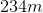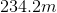Explanation:

The Law of Cosines for sideis,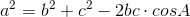.

Plugging in the information we know, the formula is,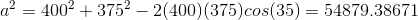.

Then take the square of both sides: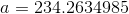.

Finally, round to the appropriate units: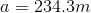.

Example Question #44 : Trigonometric Applications

Use the Law of Cosines to solve for the specified variable.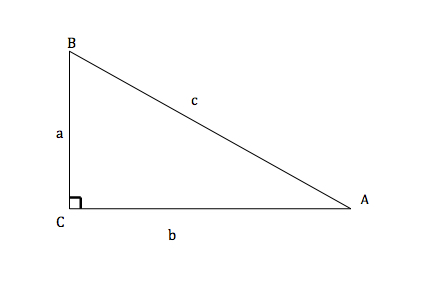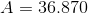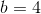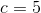Solve for. Round to the nearest tenth.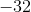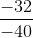None of these answers are correct.Explanation:

Law of Cosines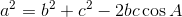Therefore...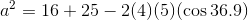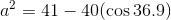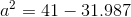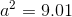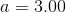After rounding...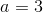All Precalculus Resources